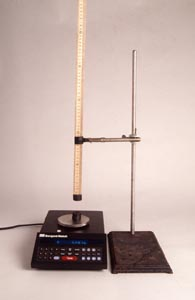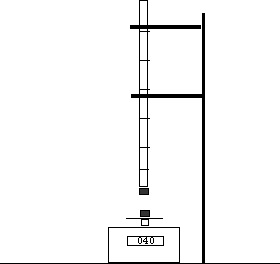The Force between Two Magnets as a Function of Distance

The force between two magnets is not necessarily inverse square.A digital scale can be used to measure the force between two magnets as a function of distance.
The upper magnet is glued to a meter stick. Click image to enlarge.

Introduction

You can use a scale with a digital readout to measure the force between two magnets as a function of distance. A plot reveals that the force does not decrease as the inverse square of the distance.

Material

A scale to measure weight (with a digital readout.)

two magnets, 1.25 inch diameter disk magnets from Radio Shack work well.

If your scale only reads to +- 0.1 gram then you will need to use neodymium magnets at least 2 cm x 2 cm x1 cm which exert larger forces on each other.

Graph paper and a calculator on which to take logs or log-log paper.

a hot melt glue gun.

A meter stick and a way to mount the meter stick over the pan of the scale, e.g. a ring stand with test tube clamps.

A metric ruler

Assembly

Tape one magnet on the pan of the scale.

Zero the scale.

Hot-melt-glue the second magnet to the end of the meter stick, measurements will be easier if you glue it near the "0" end.

Mount the meter stick so that the magnet is exactly over the meter stick on the pan of the scale. Also mount the meter stick so that it can be easily slide up-and down, varying the distance between the two magnets. Create a reference point next to the meter stick that the distance the meter stick is moved can be measured.To Do and Notice

Check that the scale reads zero when the upper magnet is removed. (Use the "Tare" option on the scale.)

Mount the magnet on the meter stick as far from the magnet on the scale as possible.

Note the reading on the scale, it should still be zero, or nearly zero.

Move the magnet on the meter stick closer to the magnet on the scale. The reading should increase if the magnets are set to repel. If the reading decreases remove the magnet from the scale and turn it over. Then start over again.

Move the magnet on the meter stick down until the reading on the scale increases.

Use the ruler to measure the distance between the centers of the two magnets. (This distance is equal to the distance between the tops of two identical magnets.)

Measure the force on the scale and record your data.

Continue to move the magnets together recording the force of their repulsion versus the distance between their centers. You should be able to devise a way of doing this with the meter stick to which one magnet is glued.

Continue measuring until the two magnets are almost touching.

Math Root Make a Graph

Plot a graph of force versus distance on regular graph paper.

Notice how the force changes with distance. The force gets weaker as the distance decreases.

Make a log-log plot of your data.

Plot a graph of force versus distance on log-log paper, or plot the log of the force versus the log of the distance on regular graph paper.

Notice that over a range of distances the data lie on a straight line on the log-log plot.

Measure the slope of the straight line. (Use a ruler to measure the rise versus the run.) The slope of the line will be near minus four.

What's Going On?

On regular graph paper the graph of force versus distance is not a straight line. However on a log-log plot it is.

A straight line on a log-log plot is produced when the dependent variable is a power law of the dependent variable. i.e. when y = a xb.

The slope of the graph of y versus x on a log-log plot gives the power, b.

If the line has a slope of 2 then y = x2. If the line has a slope of -2 then the force is y = x-2. If the force between the two magnets decreases as the inverse square then a log-log plot of force versus distance would be a straight line with a slope of -2. It is not. It is a line with a slope of -4.

If you could have magnetic monopoles, just a north pole or just a south pole, then the force between these monopoles would indeed decrease proportional to the inverse square of the distance. However magnetic monopoles have never been found in nature. Magnets always come with a pair of poles, north and south together called a magnetic dipole.

The force between two pairs of poles falls off proportional to the inverse fourth power of the distance between them. (The like poles repel and the unlike poles attract. The nearer poles exert stronger forces on each other than the more distant ones.)

So, at large distances, the force between these two magnets should fall off proportional to the inverse fourth power of distance. (Where large distance here means a distance large compared to the largest dimension of the magnet.)

These donut magnets are not tiny dipoles. When they get closer, each dipole on one magnet interacts with all the dipoles on the other, the dipoles range over many different distances at the same time. The resulting dependence of force on distance is complicated but when the distance between the magnets get small compared to the diameter the force becomes more constant with distance. The log-log plot rolls over and is no longer a straight line.

Other measurements

Measure the force between a magnet and a steel washer on the balance.

Notice that the dependence of force on distance is different. In particular the force drops off more quickly for the steel washer than it does for the magnet. The washer becomes magnetized when it is near a magnet. The magnetization of the washer falls off in proportion to the strength of the magnetic field from magnet. The magnetic field of the first magnet falls off as the inverse third power of distance, combined with the inverse fourth power fall off of the force between two magnets the resulting force falls off as the inverse seventh power. (However if the steel washer is not a perfect soft magnet, that is if it retains magnetization when removed from the presence of a magnet then the force will fall off somewhat more slowly than the inverse seventh power.)

Measure the forces between magnets with different shapes. Long rod shaped magnets have their north and south poles separated by a large distance. Over distances large compared to the diameter of these magnets and small compared to the length the force between two poles will be nearly inverse square.

Etc

The electric force between two spheres of charge decrease with distance inversely as the square of the distance. However When the charge is arranged in two long lines then the force falls off inversely proportional to distance (for distances small compared to the length of the lines.)

While if the charge is arranged in to sheets the force does not change with distance.

The same proportionalities hold for lights as well The intensity of a point of light decreases as the inverse square of distance, from a line of line inversely proportional to distance, and from a large flat plane of light the intensity does not decrease with distance.